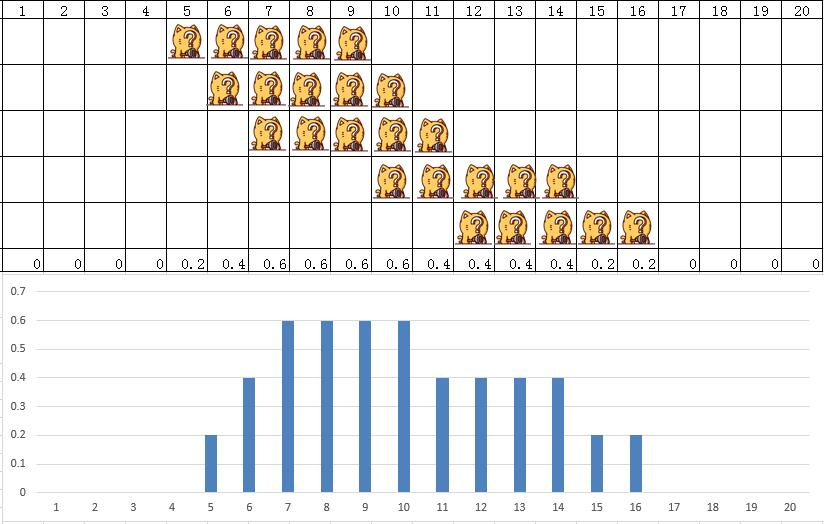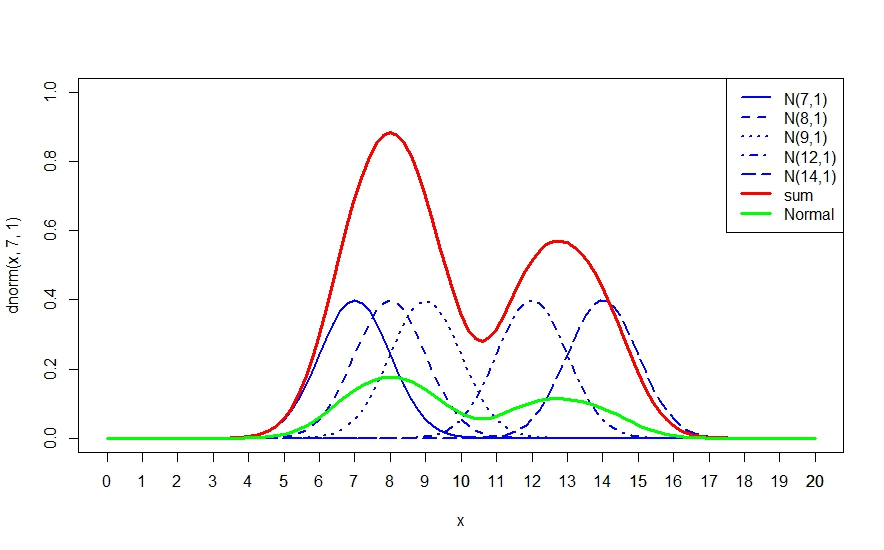# 白话空间统计二十一：密度分析（二）5个原始点的分配，每个点滑动平均5个单位，也就是每个单位的密度就是0.2，把它们有重合的地方累加起来，最后总得值也是等于5的。下面的直方图可以很明显的显示了这种方式的分布效果，但是带来的问题，依然是在不同区域的边界产生了跳跃：密度值从一个值到另外一个值仍然有突然变化的可能。当然，这种方式，密度始终围绕着原始值进行均匀散布，但是在值的变化过程中还还缺了一个中间值来进行表示。aa1 <-curve(dnorm(x, 7, 1), from = 0, to = 20,xlim=c(0,20),ylim=c(0,1.0),col=”blue”,lwd=2,lty=1) aa2 <-curve(dnorm(x, 8, 1), from = 0, to = 20,add=T,col=”blue”,lwd=2,lty=2) aa3 <-curve(dnorm(x, 9, 1), from = 0, to = 20,add=T,col=”blue”,lwd=2,lty=3) aa4 <-curve(dnorm(x, 12, 1), from = 0, to = 20,add=T,col=”blue”,lwd=2,lty=4) aa5 <-curve(dnorm(x, 14, 1), from = 01, to = 20,add=T,col=”blue”,lwd=2,lty=5) axis(side=1,at=c(1:20)) y = aa1\$y +aa2\$y+aa3\$y+aa4\$y+aa5\$y y2 = y /5 lines(aa1\$x,y,lwd=3,lty=1,col=”red”) lines(aa1\$x,y2,lwd=3,lty=1,col=”green”) legend(“topright”,        legend = c(“N(7,1)”,”N(8,1)”,”N(9,1)”,”N(12,1)”,”N(14,1)”,”sum”,”Normal”),        col=c(“blue”,”blue”,”blue”,”blue”,”blue”,”red”,”green”),        lwd=c(2,2,2,2,2,3,3),        lty=c(1,2,3,4,5,1,1))